Up: Computer Vision IT412 Previous: Image rectification

# Computing the epipolar lines

Given a point m that has coordinates (u1,v1) in the first retinal plane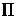,it is known that its correspondence and the fundamental matrix are related by:This equation can be expanded to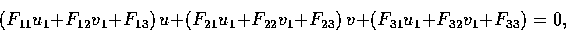where u1 and v1 are known entities, u and v are variables. This is the equation of a line in the second retinal plane. It is the epipolar line on which m' must lie.

Given a point m that has coordinates (u1,v1) in the first retinal plane,to compute the epipolar line l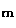that contains m, we must first compute the epipole e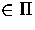.

e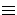intersection point of all epipolar lines in.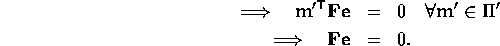• The epipole e inprojection of C' ontonull vector of F.
• The epipole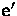in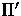projection of C ontonull vector of F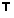.

The coordinates of lcan then be computed as the cross-product of e and m.

Robyn Owens
10/29/1997